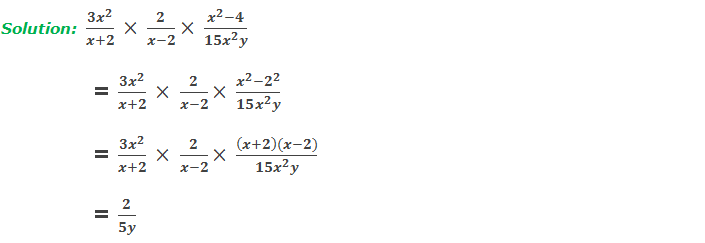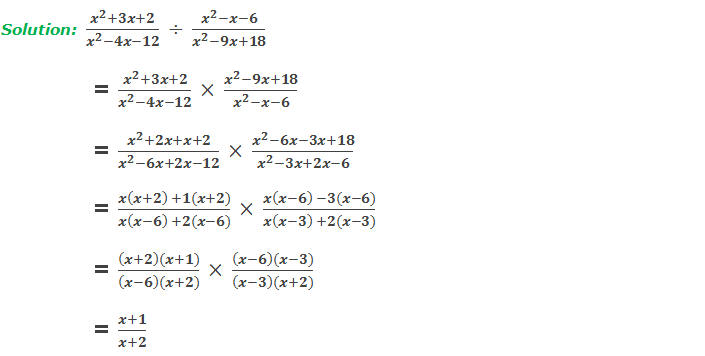# Multiplication and division of algebraic fractions

Multiplication and division of algebraic fractions

Multiplication of algebraic fractions: In the case of multiplication of monomial algebraic fractions, we should multiply the numerators and denominators separately. Then, the product is reduced to the lowest terms by using the laws of indices. For example,********************

10 Math Problems officially announces the release of Quick Math Solver, an Android App on the Google Play Store for students around the world.

********************

In the case of multiplication of polynomial algebraic fractions, the numerators and denominators are factorized. Then, they are reduced to their lowest terms. For example,

Division of algebraic fractions: In the case of division of algebraic fractions, we should multiply the dividend by the reciprocal of the divisor as like the process of multiplication of algebraic fractions. For example,

Look at the following worked out examples:

Workout ExamplesYou can comment your questions or problems regarding multiplication and division of algebraic fractions here.